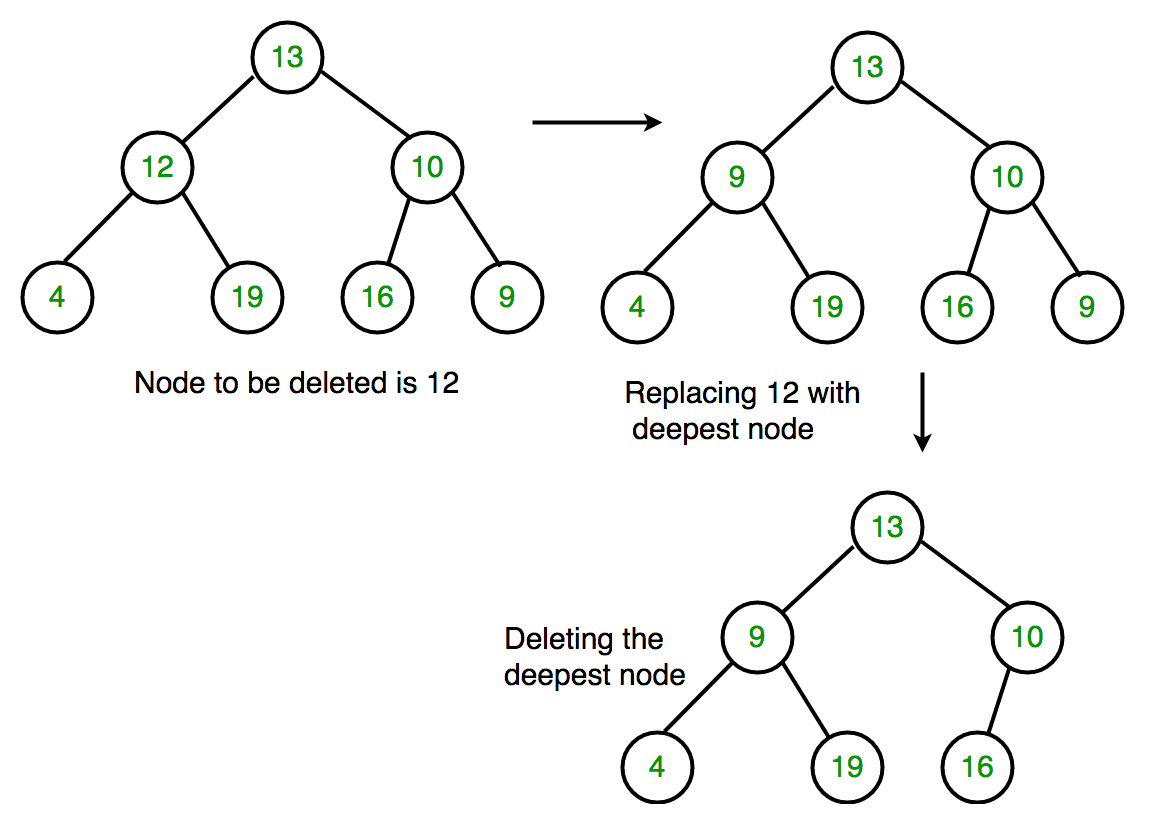Related Articles
Deletion in a Binary Tree
• Difficulty Level : Medium
• Last Updated : 31 Mar, 2021

Given a binary tree, delete a node from it by making sure that tree shrinks from the bottom (i.e. the deleted node is replaced by bottom most and rightmost node). This is different from BST deletion. Here we do not have any order among elements, so we replace with last element.
Examples :

```Delete 10 in below tree
10
/    \
20     30
Output :
30
/
20

Delete 20 in below tree
10
/    \
20     30
\
40
Output :
10
/   \
40    30   ```

Algorithm
1. Starting at root, find the deepest and rightmost node in binary tree and node which we want to delete.
2. Replace the deepest rightmost node’s data with node to be deleted.
3. Then delete the deepest rightmost node.## C++

 `// C++ program to delete element in binary tree``#include ``using` `namespace` `std;` `/* A binary tree node has key, pointer to left``child and a pointer to right child */``struct` `Node {``    ``int` `key;``    ``struct` `Node *left, *right;``};` `/* function to create a new node of tree and``return pointer */``struct` `Node* newNode(``int` `key)``{``    ``struct` `Node* temp = ``new` `Node;``    ``temp->key = key;``    ``temp->left = temp->right = NULL;``    ``return` `temp;``};` `/* Inorder traversal of a binary tree*/``void` `inorder(``struct` `Node* temp)``{``    ``if` `(!temp)``        ``return``;``    ``inorder(temp->left);``    ``cout << temp->key << ``" "``;``    ``inorder(temp->right);``}` `/* function to delete the given deepest node``(d_node) in binary tree */``void` `deletDeepest(``struct` `Node* root,``                  ``struct` `Node* d_node)``{``    ``queue<``struct` `Node*> q;``    ``q.push(root);` `    ``// Do level order traversal until last node``    ``struct` `Node* temp;``    ``while` `(!q.empty()) {``        ``temp = q.front();``        ``q.pop();``        ``if` `(temp == d_node) {``            ``temp = NULL;``            ``delete` `(d_node);``            ``return``;``        ``}``        ``if` `(temp->right) {``            ``if` `(temp->right == d_node) {``                ``temp->right = NULL;``                ``delete` `(d_node);``                ``return``;``            ``}``            ``else``                ``q.push(temp->right);``        ``}` `        ``if` `(temp->left) {``            ``if` `(temp->left == d_node) {``                ``temp->left = NULL;``                ``delete` `(d_node);``                ``return``;``            ``}``            ``else``                ``q.push(temp->left);``        ``}``    ``}``}` `/* function to delete element in binary tree */``Node* deletion(``struct` `Node* root, ``int` `key)``{``    ``if` `(root == NULL)``        ``return` `NULL;` `    ``if` `(root->left == NULL && root->right == NULL) {``        ``if` `(root->key == key)``            ``return` `NULL;``        ``else``            ``return` `root;``    ``}` `    ``queue<``struct` `Node*> q;``    ``q.push(root);` `    ``struct` `Node* temp;``    ``struct` `Node* key_node = NULL;` `    ``// Do level order traversal to find deepest``    ``// node(temp) and node to be deleted (key_node)``    ``while` `(!q.empty()) {``        ``temp = q.front();``        ``q.pop();` `        ``if` `(temp->key == key)``            ``key_node = temp;` `        ``if` `(temp->left)``            ``q.push(temp->left);` `        ``if` `(temp->right)``            ``q.push(temp->right);``    ``}` `    ``if` `(key_node != NULL) {``        ``int` `x = temp->key;``        ``deletDeepest(root, temp);``        ``key_node->key = x;``    ``}``    ``return` `root;``}` `// Driver code``int` `main()``{``    ``struct` `Node* root = newNode(10);``    ``root->left = newNode(11);``    ``root->left->left = newNode(7);``    ``root->left->right = newNode(12);``    ``root->right = newNode(9);``    ``root->right->left = newNode(15);``    ``root->right->right = newNode(8);` `    ``cout << ``"Inorder traversal before deletion : "``;``    ``inorder(root);` `    ``int` `key = 11;``    ``root = deletion(root, key);` `    ``cout << endl;``    ``cout << ``"Inorder traversal after deletion : "``;``    ``inorder(root);` `    ``return` `0;``}`

## Java

 `// Java program to delete element``// in binary tree``import` `java.util.LinkedList;``import` `java.util.Queue;` `class` `GFG{``    ` `// A binary tree node has key, pointer to``// left child and a pointer to right child``static` `class` `Node``{``    ``int` `key;``    ``Node left, right;``    ` `    ``// Constructor``    ``Node(``int` `key)``    ``{``        ``this``.key = key;``        ``left = ``null``;``        ``right = ``null``;``    ``}``}` `static` `Node root;``static` `Node temp = root;` `// Inorder traversal of a binary tree``static` `void` `inorder(Node temp)``{``    ``if` `(temp == ``null``)``        ``return``;` `    ``inorder(temp.left);``    ``System.out.print(temp.key + ``" "``);``    ``inorder(temp.right);``}` `// Function to delete deepest``// element in binary tree``static` `void` `deleteDeepest(Node root,``                          ``Node delNode)``{``    ``Queue q = ``new` `LinkedList();``    ``q.add(root);``    ` `    ``Node temp = ``null``;``    ` `    ``// Do level order traversal until last node``    ``while` `(!q.isEmpty())``    ``{``        ``temp = q.peek();``        ``q.remove();``        ` `        ``if` `(temp == delNode)``        ``{``            ``temp = ``null``;``            ``return``;``            ` `        ``}``        ``if` `(temp.right!=``null``)``        ``{``            ``if` `(temp.right == delNode)``            ``{``                ``temp.right = ``null``;``                ``return``;``        ``}``        ``else``            ``q.add(temp.right);``    ``}` `    ``if` `(temp.left != ``null``)``    ``{``        ``if` `(temp.left == delNode)``        ``{``            ``temp.left = ``null``;``            ``return``;``        ``}``        ``else``            ``q.add(temp.left);``    ``}``}``}` `// Function to delete given element``// in binary tree``static` `void` `delete(Node root, ``int` `key)``{``    ``if` `(root == ``null``)``        ``return``;``        ` `    ``if` `(root.left == ``null` `&&``       ``root.right == ``null``)``    ``{``        ``if` `(root.key == key)``        ``{``              ``root=``null``;``              ``return``;``        ``}``        ``else``            ``return``;``    ``}``    ` `    ``Queue q = ``new` `LinkedList();``    ``q.add(root);``    ``Node temp = ``null``, keyNode = ``null``;``    ` `    ``// Do level order traversal until``    ``// we find key and last node.``    ``while` `(!q.isEmpty())``    ``{``        ``temp = q.peek();``        ``q.remove();``        ` `        ``if` `(temp.key == key)``            ``keyNode = temp;` `        ``if` `(temp.left != ``null``)``            ``q.add(temp.left);` `        ``if` `(temp.right != ``null``)``            ``q.add(temp.right);``    ``}` `    ``if` `(keyNode != ``null``)``    ``{``        ``int` `x = temp.key;``        ``deleteDeepest(root, temp);``        ``keyNode.key = x;``    ``}``}` `// Driver code``public` `static` `void` `main(String args[])``{``    ``root = ``new` `Node(``10``);``    ``root.left = ``new` `Node(``11``);``    ``root.left.left = ``new` `Node(``7``);``    ``root.left.right = ``new` `Node(``12``);``    ``root.right = ``new` `Node(``9``);``    ``root.right.left = ``new` `Node(``15``);``    ``root.right.right = ``new` `Node(``8``);` `    ``System.out.print(``"Inorder traversal "` `+``                     ``"before deletion:"``);``    ``inorder(root);` `    ``int` `key = ``11``;``    ``delete(root, key);` `    ``System.out.print(``"\nInorder traversal "` `+``                     ``"after deletion:"``);``    ``inorder(root);``}``}` `// This code is contributed by Ravi Kant Verma`

## Python3

 `# Python3 program to illustrate deletion in a Binary Tree`` ` `# class to create a node with data, left child and right child.``class` `Node:``    ``def` `__init__(``self``,data):``        ``self``.data ``=` `data``        ``self``.left ``=` `None``        ``self``.right ``=` `None`` ` `# Inorder traversal of a binary tree``def` `inorder(temp):``    ``if``(``not` `temp):``        ``return``    ``inorder(temp.left)``    ``print``(temp.data, end ``=` `" "``)``    ``inorder(temp.right)`` ` `# function to delete the given deepest node (d_node) in binary tree``def` `deleteDeepest(root,d_node):``    ``q ``=` `[]``    ``q.append(root)``    ``while``(``len``(q)):``        ``temp ``=` `q.pop(``0``)``        ``if` `temp ``is` `d_node:``            ``temp ``=` `None``            ``return``        ``if` `temp.right:``            ``if` `temp.right ``is` `d_node:``                ``temp.right ``=` `None``                ``return``            ``else``:``                ``q.append(temp.right)``        ``if` `temp.left:``            ``if` `temp.left ``is` `d_node:``                ``temp.left ``=` `None``                ``return``            ``else``:``                ``q.append(temp.left)`` ` `# function to delete element in binary tree``def` `deletion(root, key):``    ``if` `root ``=``=` `None` `:``        ``return` `None``    ``if` `root.left ``=``=` `None` `and` `root.right ``=``=` `None``:``        ``if` `root.key ``=``=` `key :``            ``return` `None``        ``else` `:``            ``return` `root``    ``key_node ``=` `None``    ``q ``=` `[]``    ``q.append(root)``    ``while``(``len``(q)):``        ``temp ``=` `q.pop(``0``)``        ``if` `temp.data ``=``=` `key:``            ``key_node ``=` `temp``        ``if` `temp.left:``            ``q.append(temp.left)``        ``if` `temp.right:``            ``q.append(temp.right)``    ``if` `key_node :``        ``x ``=` `temp.data``        ``deleteDeepest(root,temp)``        ``key_node.data ``=` `x``    ``return` `root`` ` `# Driver code``if` `__name__``=``=``'__main__'``:``    ``root ``=` `Node(``10``)``    ``root.left ``=` `Node(``11``)``    ``root.left.left ``=` `Node(``7``)``    ``root.left.right ``=` `Node(``12``)``    ``root.right ``=` `Node(``9``)``    ``root.right.left ``=` `Node(``15``)``    ``root.right.right ``=` `Node(``8``)``    ``print``(``"The tree before the deletion:"``)``    ``inorder(root)``    ``key ``=` `11``    ``root ``=` `deletion(root, key)``    ``print``()``    ``print``(``"The tree after the deletion;"``)``    ``inorder(root)``     ` `# This code is contributed by Monika Anandan`
Output
```Inorder traversal before deletion : 7 11 12 10 15 9 8
Inorder traversal after deletion : 7 8 12 10 15 9 ```

Note: We can also replace node’s data that is to be deleted with any node whose left and right child points to NULL but we only use deepest node in order to maintain the Balance of a binary tree.
This article is contributed by Yash Singla. If you like GeeksforGeeks and would like to contribute, you can also write an article using contribute.geeksforgeeks.org or mail your article to contribute@geeksforgeeks.org. See your article appearing on the GeeksforGeeks main page and help other Geeks.
Please write comments if you find anything incorrect, or you want to share more information about the topic discussed above.

Attention reader! Don’t stop learning now. Get hold of all the important DSA concepts with the DSA Self Paced Course at a student-friendly price and become industry ready.

My Personal Notes arrow_drop_up